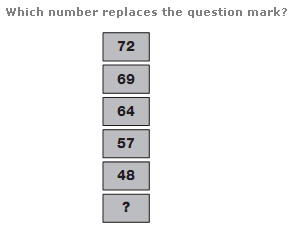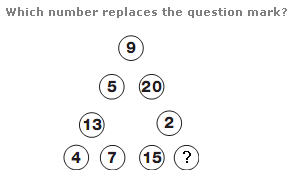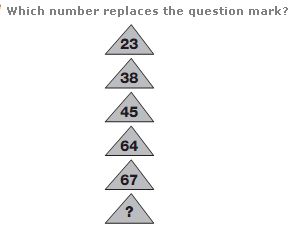# Puzzles - Number puzzles

### Exercise :: Number puzzlesAnswer : 37 Explanation : As you move down, subtract 3 from the previous number, then 5, 7, 9 and 11.Answer : 11 Explanation : Taking any side of the triangle, the sum of the two corner digits is written in one of the circles on that side, and the difference is written in the other circle.Answer : 90 Explanation : As you move down, the numbers represent multiples of 12, subtracting 1, for the first step, adding 2 for the second, subtracting 3 for the third, adding 4 for the next etc. etc.